Custom

# Temperature to Frequency Conversion using GreenPAK™October 15, 2021 by GreenPAK™ Family of Integrated Circuits
Share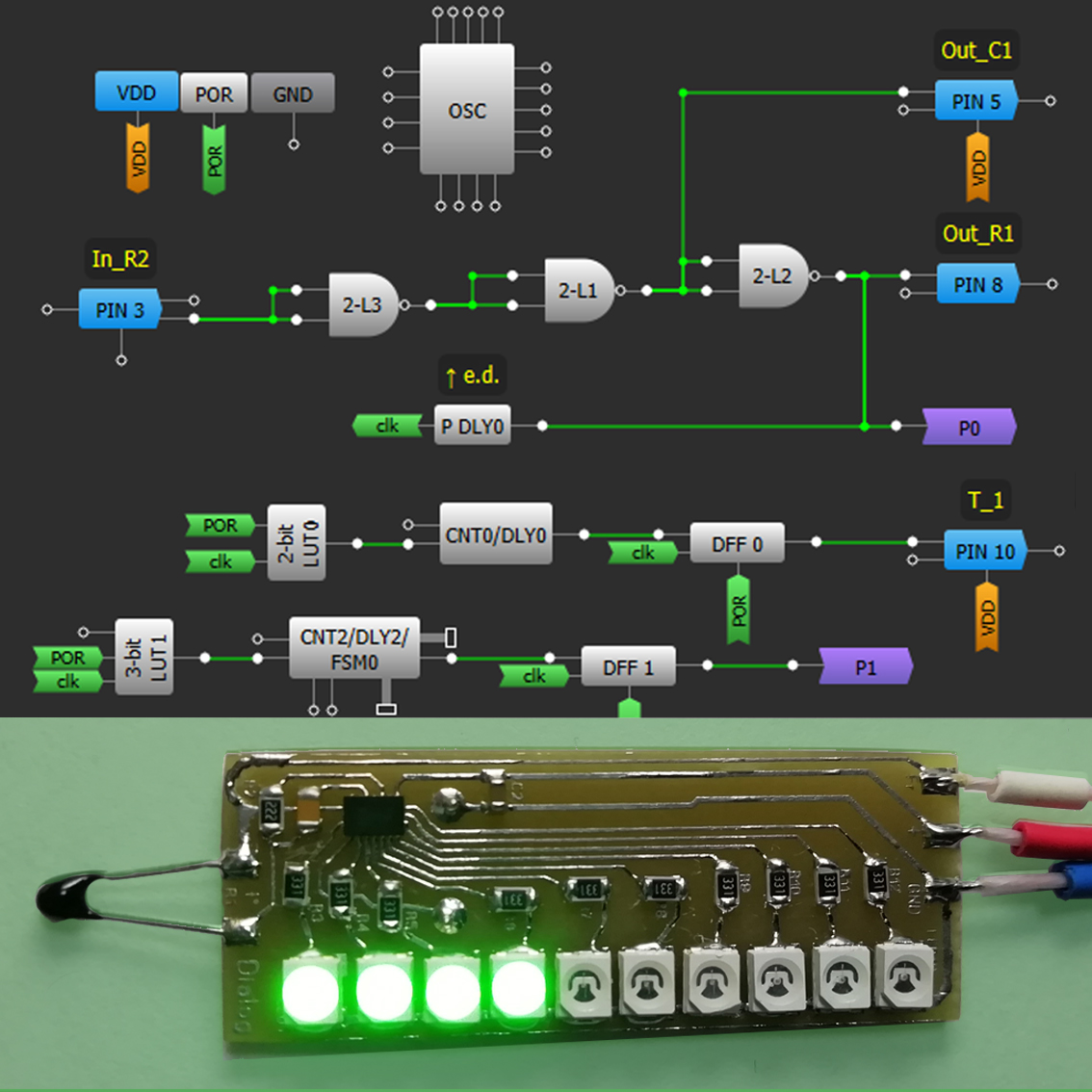Discover a simplified technique for direct converting a temperature sensor analog signal to digital frequency.

## Hardware

 1 SLG4DVKADV 1 SLG46108V-SKT 1 SLG46620V-SKT

Temperature sensors are one of the most important kinds of physical sensors, because many different processes (in everyday life as well) are regulated by temperature. Besides, the temperature measurement allows indirect determination of other physical parameters, such as matter flow rate, fluid level, etc. Typically, sensors convert the measured physical value into an analog signal, and temperature sensors are no exception here. For processing by the CPU or computer, the analog temperature signal must be converted into a digital form. For such a conversion expensive analog-to-digital converters (ADCs) are commonly used.

The purpose of this project is to develop and present a simplified technique for direct conversion of the analog signal from a temperature sensor into a digital signal with proportional frequency using GreenPAK. Subsequently, the frequency of a digital signal that varies depending on temperature can then be more easily measured with a fairly high accuracy and then converted to the required units of measurement. Such direct transformation is interesting in the first place by the fact that there is no need for the use of expensive analog-to-digital converters. Also, digital signal transmission is more reliable than analog.

Below we described steps needed to understand how the solution has been programmed to create the temperature-to-frequency converter. However, if you just want to get the result of programming, download GreenPAK Designer software to view the already completed GreenPAK Design file. Plug the GreenPAK Development Kit into your computer and hit the program to design the device.

## Design and Circuit Analysis

Different types of temperature sensors and their signal processing circuits can be used depending on specific requirements, primarily in temperature range, and accuracy. The most widely used are NTC thermistors, which reduce the value of their electrical resistance with increasing temperature (see Figure 1). They have a significantly higher temperature coefficient of resistance compared to metal resistive sensors (RTDs) and they cost much less. The main disadvantage of thermistors is their nonlinear dependence of the characteristic "resistance vs. temperature". In our case, this does not play a significant role since during conversion, there is an exact correspondence of the frequency to thermistor resistance, and therefore, the temperature.Figure 1 shows the graphical dependence of thermistor resistance vs temperature (which were taken from manufacturer datasheets). For our design, we used two similar NTC thermistors with a typical resistance of 10 kOhm at 25Â°C.The basic idea of the direct transformation of the temperature signal into the output digital signal of a proportional frequency is the use of the thermistor R1 together with the capacitor C1 in the frequency-setting R1C1-circuit of the generator, as part of a classical ring oscillator using three â€œNANDâ€ logic elements. The time constant of R1C1 depends on the temperature, because when the temperature changes, the thermistor's resistance will change accordingly.

The frequency of the output digital signal can be calculated using the following formula (1):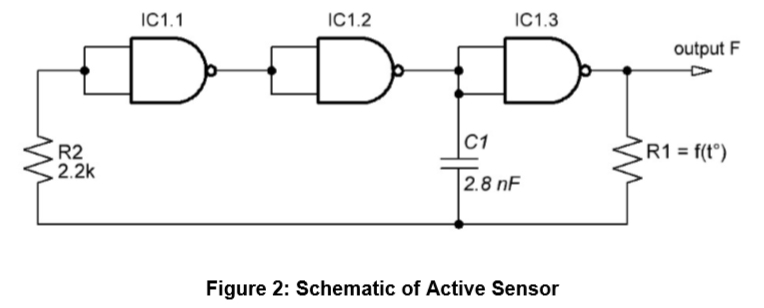This type of oscillator typically adds a resistor R2 to limit the current through the input diodes and reduce the load on the input elements of the circuit. If the resistance value of R2 is much smaller than the resistance of R1, then it does not actually affect the generation frequency.

Consequently, based on the GreenPAK SLG46108V, two variants of the temperature to frequency converter were constructed (see Figure 5). The application circuit of these sensors is presented in Figure 3.The design, as we have already said, is quite simple, it is a chain of three NAND elements that form a ring oscillator (see Figure 4 and Figure 2) with one digital input (PIN#3), and two digital outputs (PIN#6 and PIN#8) for connection to external circuitry.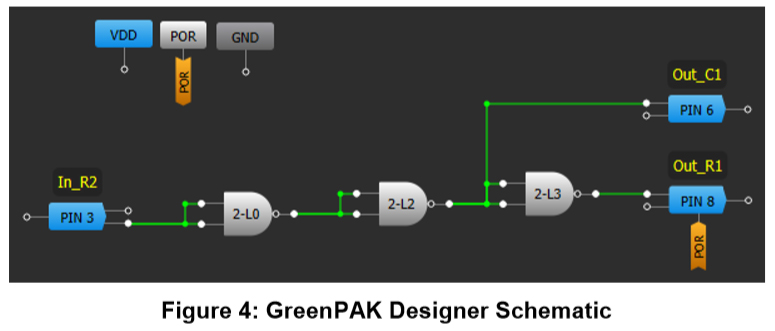Figure 5 Photo shows the active temperature sensors (a one cent coin is for scale).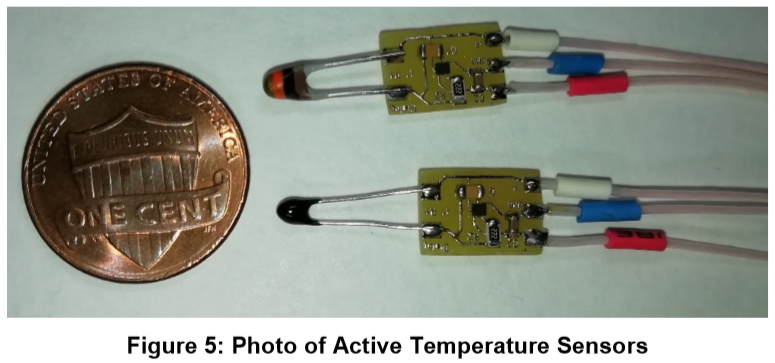Measurements were made to evaluate the correct function of these active temperature sensors. Our temperature sensor was placed in a controlled chamber, the temperature inside of which could be changed to an accuracy of 0.5 Â°Ð¡. The frequency of the output digital signal was recorded and the results are presented in Figure 6.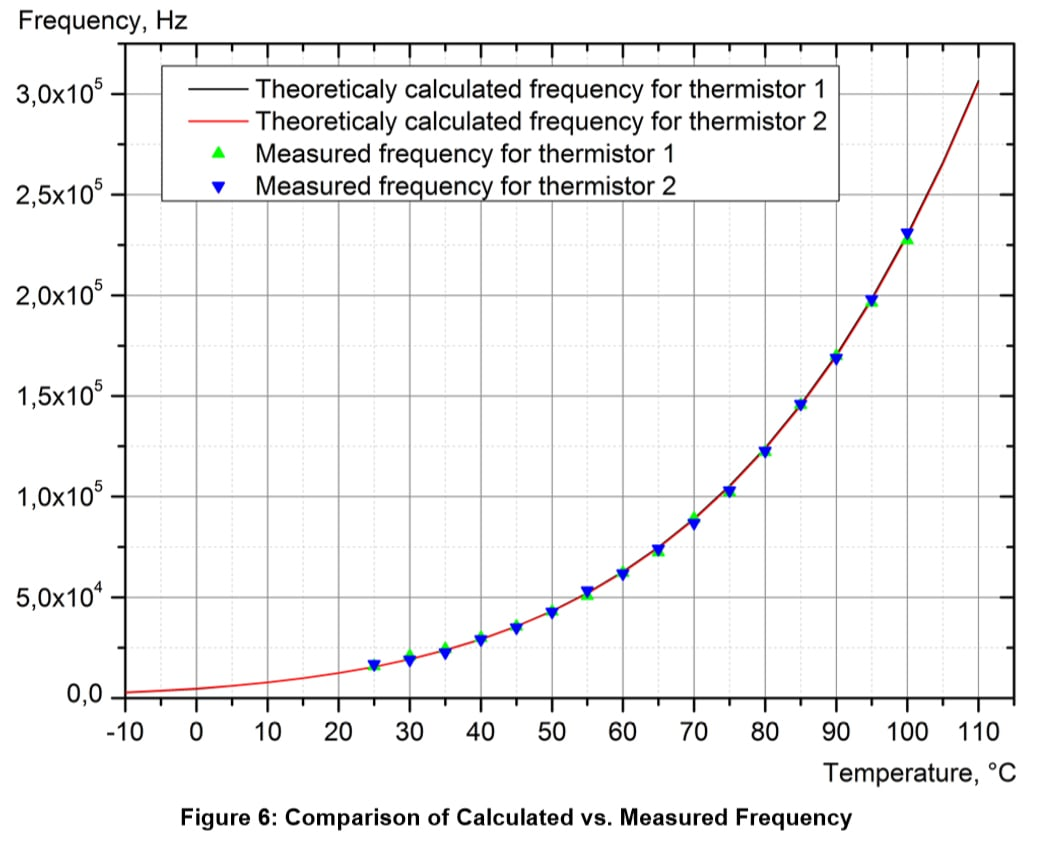As can be seen from the plot shown, the frequency measurements (green and blue triangles) almost completely coincide with the theoretical values (black and red lines) according to formula (1) given above. Consequently, this method of converting temperature to frequency is working correctly.

Also, a third active temperature sensor was built (see Figure 7) to demonstrate the possibility of simple processing with visible temperature indication. Using the GreenPAK SLG46620V, which contains 10 delay elements, we have built ten frequency detectors (see Figure 9), each of which is configured to detect a signal of one particular frequency. In this way, we constructed a simple thermometer with ten customizable points of indication.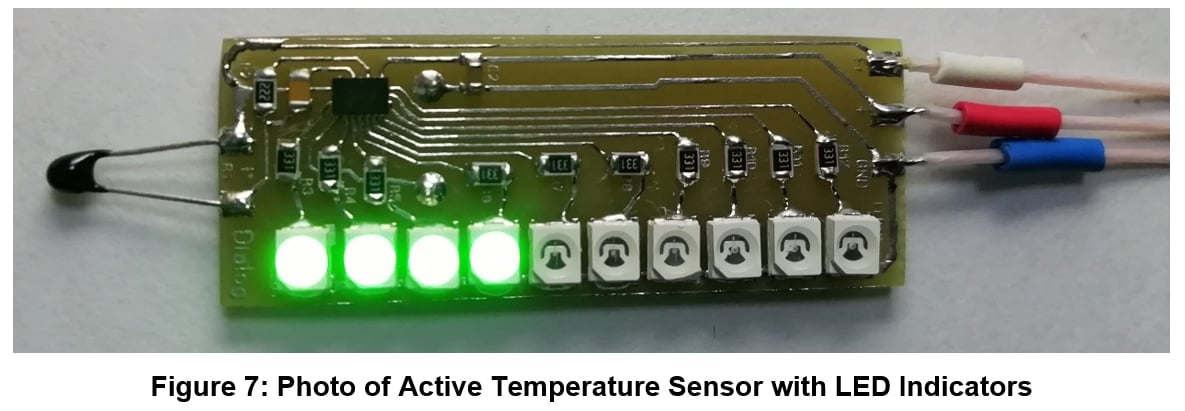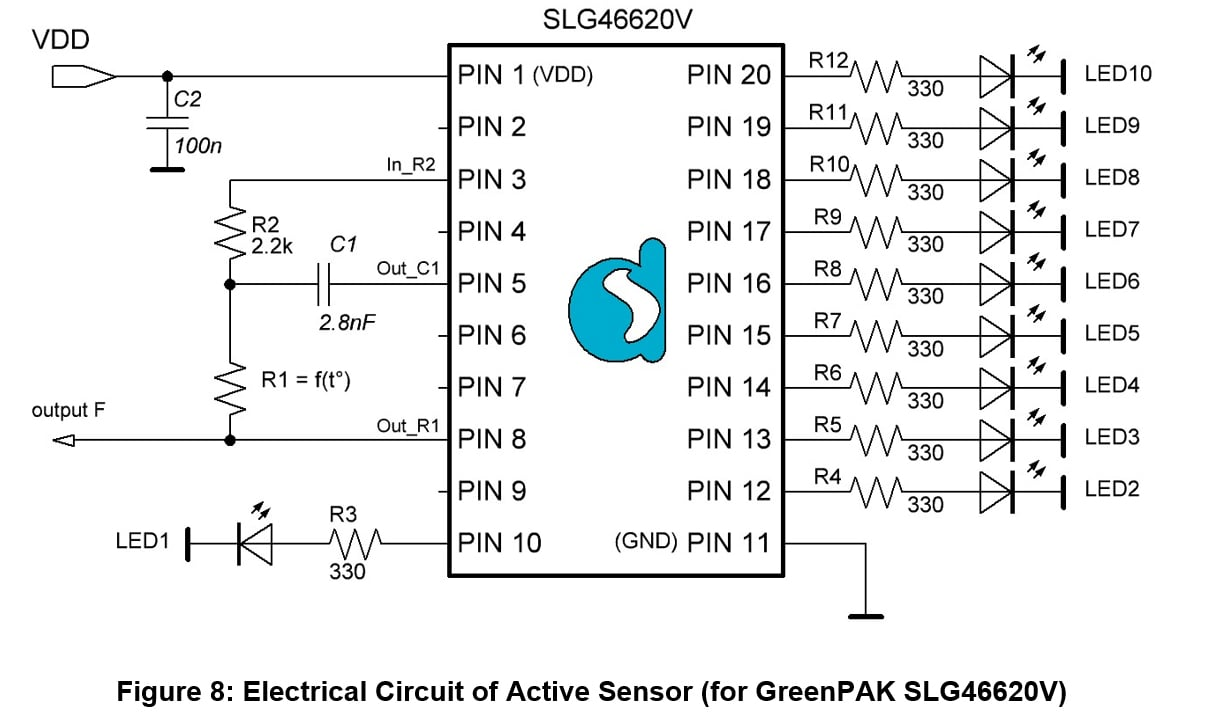Figure 8 shows the top level schematic of the active sensor with display indicators for ten temperature points. This additional function is convenient because it is possible to visually estimate temperature value without separately analyzing the generated digital signal.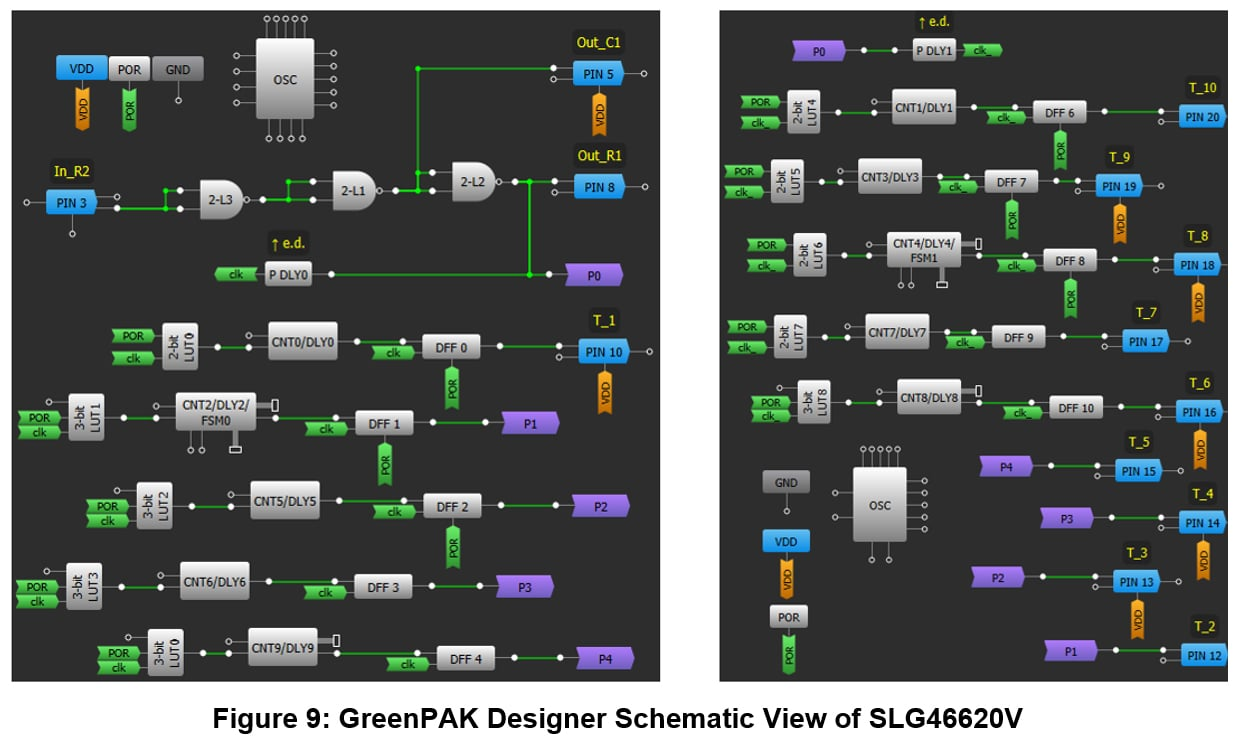## Conclusions

In this project, we proposed a method for converting a temperature sensor analog signal into a frequency modulated digital signal using GreenPAK products from Dialog. The use of thermistors in conjunction with GreenPAK allows predictable measurements without the use of expensive analog-to-digital converters, and avoiding the requirement to measure the analog signals. GreenPAK is the ideal solution for the development of this type of customizable sensor, as shown in the prototype examples constructed and tested. GreenPAK contains a large number of functional elements and circuit blocks necessary for the implementation of various circuit solutions, and this greatly reduces the number of external components of the final application circuit. Low power consumption, small chip size and low cost are an added bonus for choosing GreenPAK as the main controller for many circuit designs.